Like   Tweet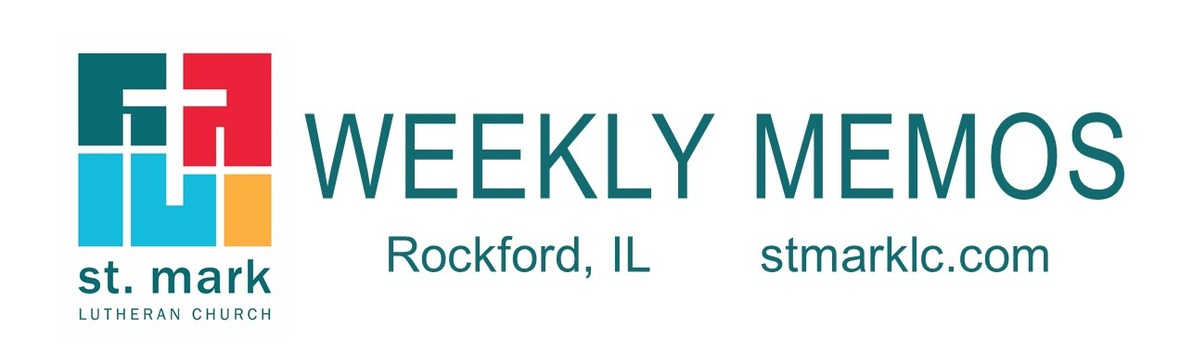## Pastoral Reflection

 table div table+table+table div table{width:100%;padding:0}table div table+table+table div table img{width:96.23%;padding:0;float:none}table div table+table+table div table td{width:100%;padding:0 1.88% 18px}/* styles */More Opportunities to Worship
Pastor Chad McKenna

We are pleased to announce the addition of a second service on Sundays, beginning in May! Please read this important letter from Pastor Chad to learn about this exciting change, and what it means for our Sunday morning schedule. Read more.

 table div table+table+table+table+table div table{width:100%;padding:0}table div table+table+table+table+table div table img{width:96.23%;padding:0;float:none}table div table+table+table+table+table div table td{width:100%;padding:0 1.88% 18px}/* styles */## Sunday, April 11

9:30 A.M. Outdoor Live-stream Worship Service
11:00 A.M. Remote Sunday School

## Monday, April 12

5:00 P.M. Children's Ministry

## Tuesday, April 13

9:00 A.M. Staff Meeting
11:30 A.M. St. Mark Duplicate Bridge - in the cafe

## Wednesday, April 14

9:00 A.M. Women's Bible Study
9:00 A.M. Men's Bible Study
9:30 A.M. St. Mark Ringers
12:00 P.M. Miriam Circle at Stockholm Inn
4:00 P.M. Outreach Ministry
7:00 P.M. Confirmation
7:00 P.M. Voices of St. Mark

## Thursday, April 15

6:30 P.M. Emotions Anonymous
6:30 P.M. Blue Lights

## Sunday, April 18

9:30 A.M. Outdoor Parking Lot Live-Stream Service
11:00 A.M. Remote Sunday School

 table div table+table+table+table+table+table+table+table div table{width:100%;padding:0}table div table+table+table+table+table+table+table+table div table img{width:96.23%;padding:0;float:none}table div table+table+table+table+table+table+table+table div table td{width:100%;padding:0 1.88% 18px}/* styles */## News and Events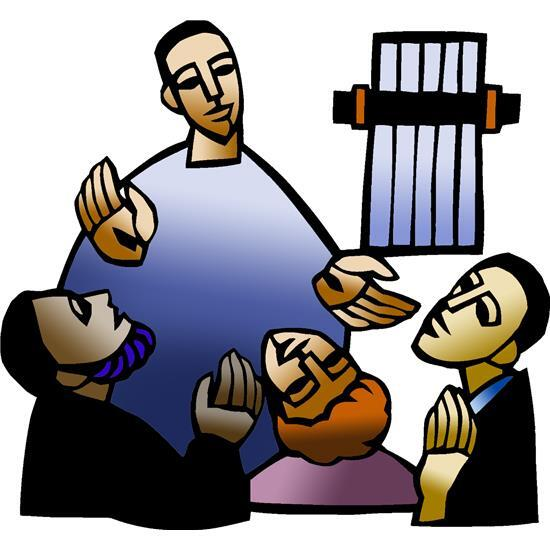Second Sunday after Easter
Join us this Sunday, April 11 outdoors at 9:30 a.m. in the parking lot for live-stream worship service. If you are unable to be here, the service will be live-streamed at this link.

 table div table+table+table+table+table+table+table+table+table+table+table div table{width:100%;padding:0}table div table+table+table+table+table+table+table+table+table+table+table div table img{width:96.23%;padding:0;float:none}table div table+table+table+table+table+table+table+table+table+table+table div table td{width:100%;padding:0 1.88% 18px}/* styles */Come Pick Up Your Easter Lily
Thank you to those who purchased lilies this year to help decorate our outdoor worship space on Easter, it looked beautiful. You may pick up your lily Friday from 9:00 - 1:00 p.m. or next week during office hours.

 table div table+table+table+table+table+table+table+table+table+table+table+table+table div table{width:100%;padding:0}table div table+table+table+table+table+table+table+table+table+table+table+table+table div table img{width:96.23%;padding:0;float:none}table div table+table+table+table+table+table+table+table+table+table+table+table+table div table td{width:100%;padding:0 1.88% 18px}/* styles */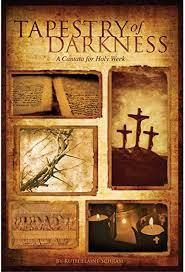Good Friday's Cantata - Tapestry of Darkness
If you haven't had a chance to watch the Cantata yet, you can enjoy this beautifully sung service that reflects upon the events of our Savior's last hours on earth by clicking this link. We pray that this service will provide a meaningful experience for you.

 table div table+table+table+table+table+table+table+table+table+table+table+table+table+table+table div table{width:100%;padding:0}table div table+table+table+table+table+table+table+table+table+table+table+table+table+table+table div table img{width:96.23%;padding:0;float:none}table div table+table+table+table+table+table+table+table+table+table+table+table+table+table+table div table td{width:100%;padding:0 1.88% 18px}/* styles */Birthday in a Bag!
To celebrate 60 years of St. Mark for the month of April, the Children’s Ministry Committee is collecting donations of 60 or more birthday kits for the local food pantries! The supplies recommended are cake mix & frosting, candles, sprinkles, small candies, or other cake decorations, festive plates & napkins, balloons, streamers, or other party decorations or any other items your family loves to use for joyful celebrations. You may drop them off at the church anytime during office hours. There will be a bin inside the south doors labeled Birthday in a Bag!

 table div table+table+table+table+table+table+table+table+table+table+table+table+table+table+table+table+table div table{width:100%;padding:0}table div table+table+table+table+table+table+table+table+table+table+table+table+table+table+table+table+table div table img{width:96.23%;padding:0;float:none}table div table+table+table+table+table+table+table+table+table+table+table+table+table+table+table+table+table div table td{width:100%;padding:0 1.88% 18px}/* styles */Thank you for participating in the ELCA Good Gifts, Global Barnyard. St. Mark raised \$1,950.00 Your gifts will help support projects in more than 60 countries around the world
Including the United States. We especially were able to raise money for a cow, a goat, a pig, honey bees, sheep, and chicks. Many of these projects help provide livestock, tools, and training for farm families. Thank you and God bless.

 table div table+table+table+table+table+table+table+table+table+table+table+table+table+table+table+table+table+table+table div table{width:100%;padding:0}table div table+table+table+table+table+table+table+table+table+table+table+table+table+table+table+table+table+table+table div table img{width:96.23%;padding:0;float:none}table div table+table+table+table+table+table+table+table+table+table+table+table+table+table+table+table+table+table+table div table td{width:100%;padding:0 1.88% 18px}/* styles */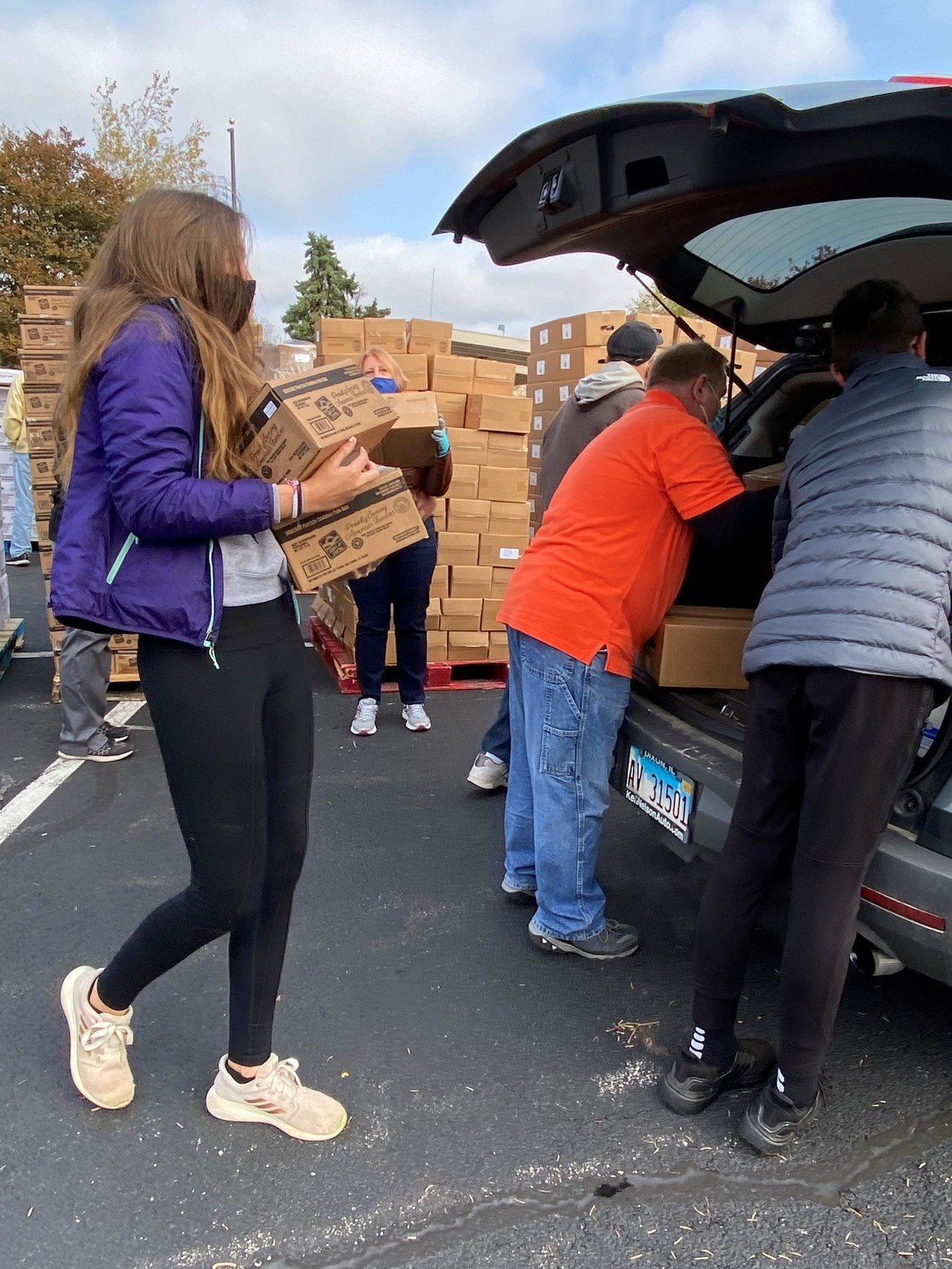Mobile Pantry Volunteers, We need YOU!
Please sign-up by calling the church office.
Upcoming dates are Saturday, April 24, 9-11:30 A.M.,
and June 26th. Volunteers are needed, can you help?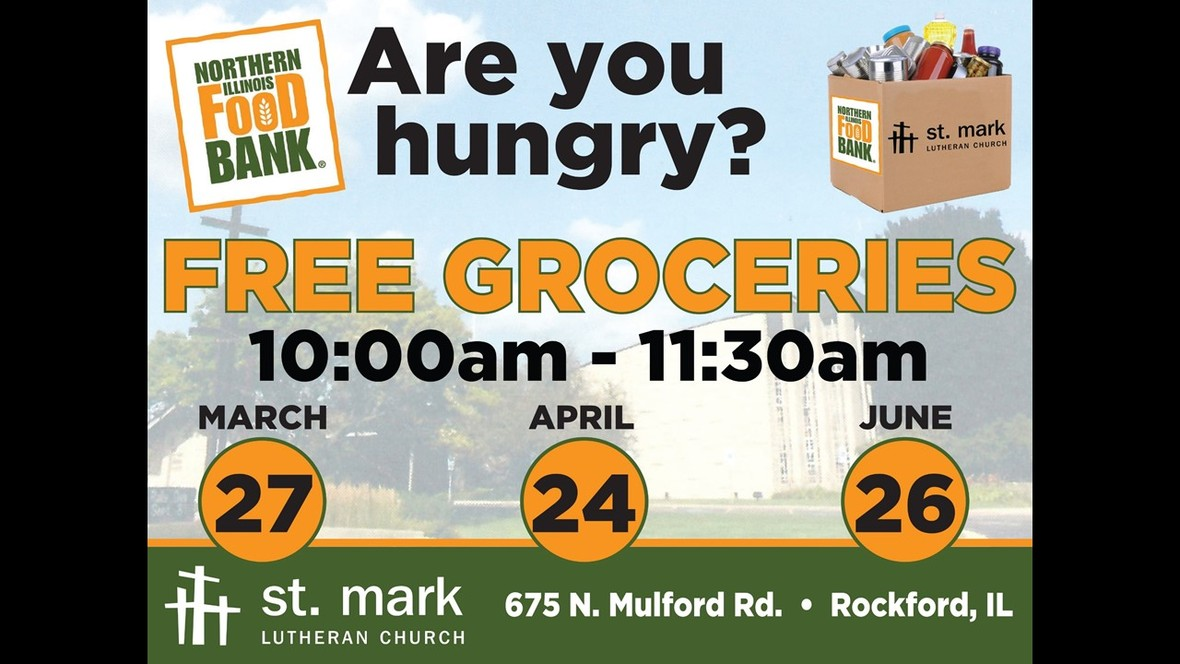table div table+table+table+table+table+table+table+table+table+table+table+table+table+table+table+table+table+table+table+table+table+table div table{width:100%;padding:0}table div table+table+table+table+table+table+table+table+table+table+table+table+table+table+table+table+table+table+table+table+table+table div table img{width:96.23%;padding:0;float:none}table div table+table+table+table+table+table+table+table+table+table+table+table+table+table+table+table+table+table+table+table+table+table div table td{width:100%;padding:0 1.88% 18px}/* styles */Summer Fun…and Service at St. Mark!
Youth 8th grade and entering high school through 12th grade are invited for summer mission days.
There will be elements of service, mission, fellowship, worship & fun together!
Save the Date and say you’ll come June 23 thru June 27th. 9:00-3:30 daily, the cost is \$50.
Text or email Melinda Alekna 815-871-0390 melinda@stmarklc.com

Yes together! Right here at St. Mark and out in the community. Participate in all five days or as you are able. The cost is just \$50! Questions? Text or e-mail 815-871-0390, melinda@stmark.com
Jesus said to them again, “Peace be with you. As the Father has sent me, so I send you.” John 20:21

 table div table+table+table+table+table+table+table+table+table+table+table+table+table+table+table+table+table+table+table+table+table+table+table+table div table{width:100%;padding:0}table div table+table+table+table+table+table+table+table+table+table+table+table+table+table+table+table+table+table+table+table+table+table+table+table div table img{width:96.23%;padding:0;float:none}table div table+table+table+table+table+table+table+table+table+table+table+table+table+table+table+table+table+table+table+table+table+table+table+table div table td{width:100%;padding:0 1.88% 18px}/* styles */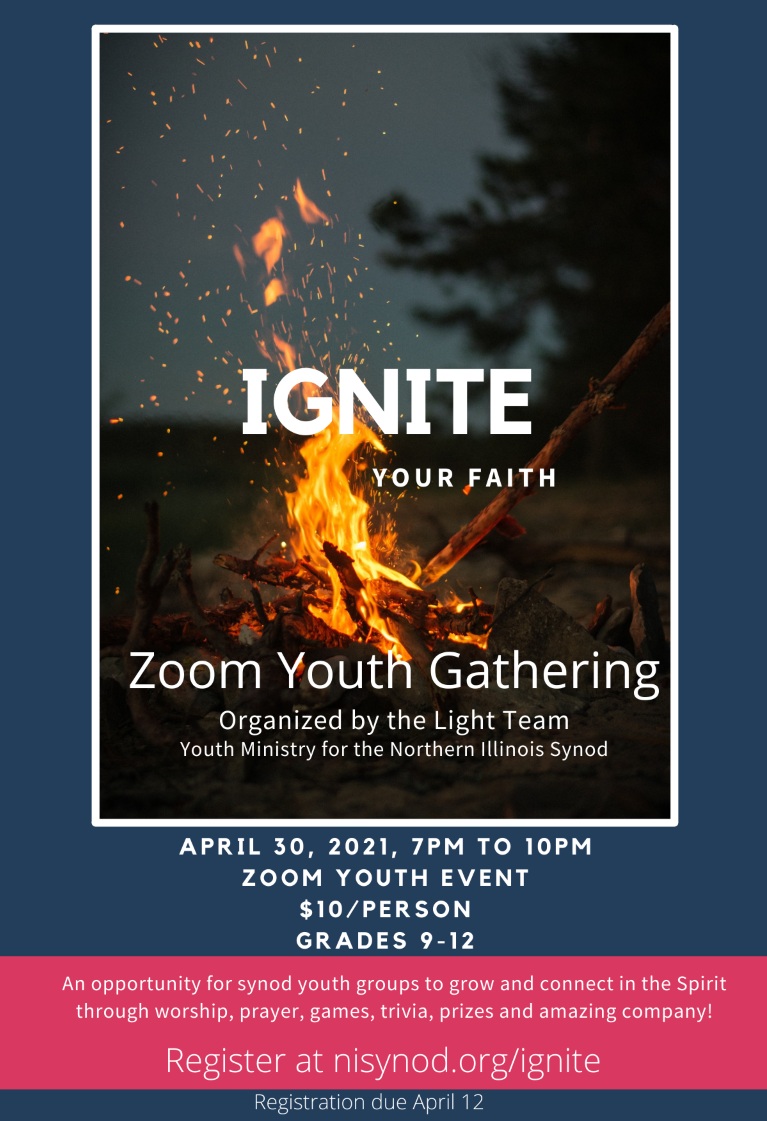Ignite is happening Friday, April 30. Join us with youth from all over the Northern Illinois Synod. Please message Melinda Alekna 815-871-0390 for details.

 table div table+table+table+table+table+table+table+table+table+table+table+table+table+table+table+table+table+table+table+table+table+table+table+table+table+table div table{width:100%;padding:0}table div table+table+table+table+table+table+table+table+table+table+table+table+table+table+table+table+table+table+table+table+table+table+table+table+table+table div table img{width:96.23%;padding:0;float:none}table div table+table+table+table+table+table+table+table+table+table+table+table+table+table+table+table+table+table+table+table+table+table+table+table+table+table div table td{width:100%;padding:0 1.88% 18px}/* styles *//* styles */ Thank you families and volunteers that helped make our Easter family event happen. God’s Re-Creation was beautiful, meaningful, well attended and fun! Families enjoyed being together, outside in the sunshine. There were learning opportunities about prayer, nature, art, story, science, and service.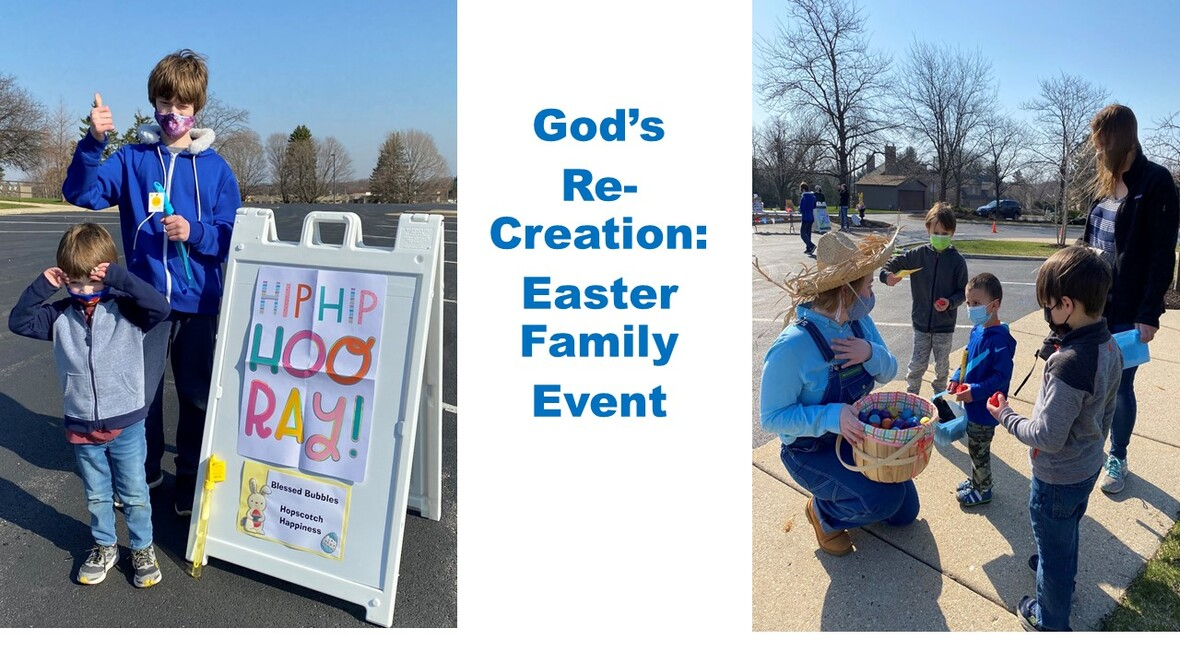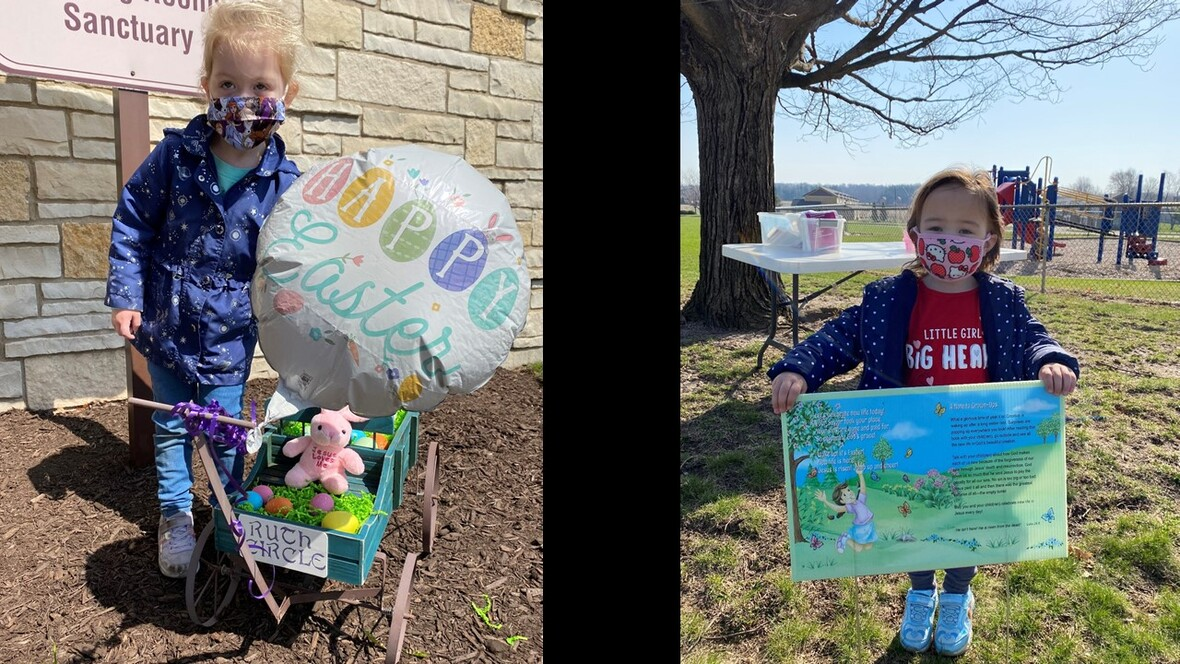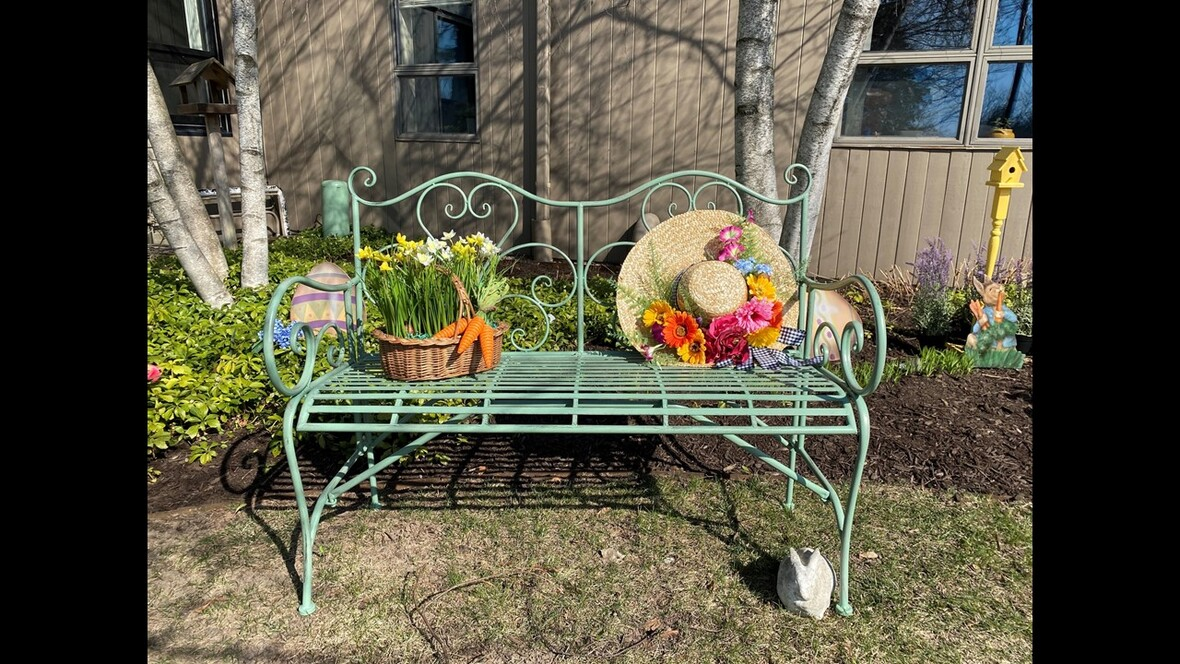table div table+table+table+table+table+table+table+table+table+table+table+table+table+table+table+table+table+table+table+table+table+table+table+table+table+table+table+table+table+table+table+table div table{width:100%;padding:0}table div table+table+table+table+table+table+table+table+table+table+table+table+table+table+table+table+table+table+table+table+table+table+table+table+table+table+table+table+table+table+table+table div table img{width:96.23%;padding:0;float:none}table div table+table+table+table+table+table+table+table+table+table+table+table+table+table+table+table+table+table+table+table+table+table+table+table+table+table+table+table+table+table+table+table div table td{width:100%;padding:0 1.88% 18px}/* styles */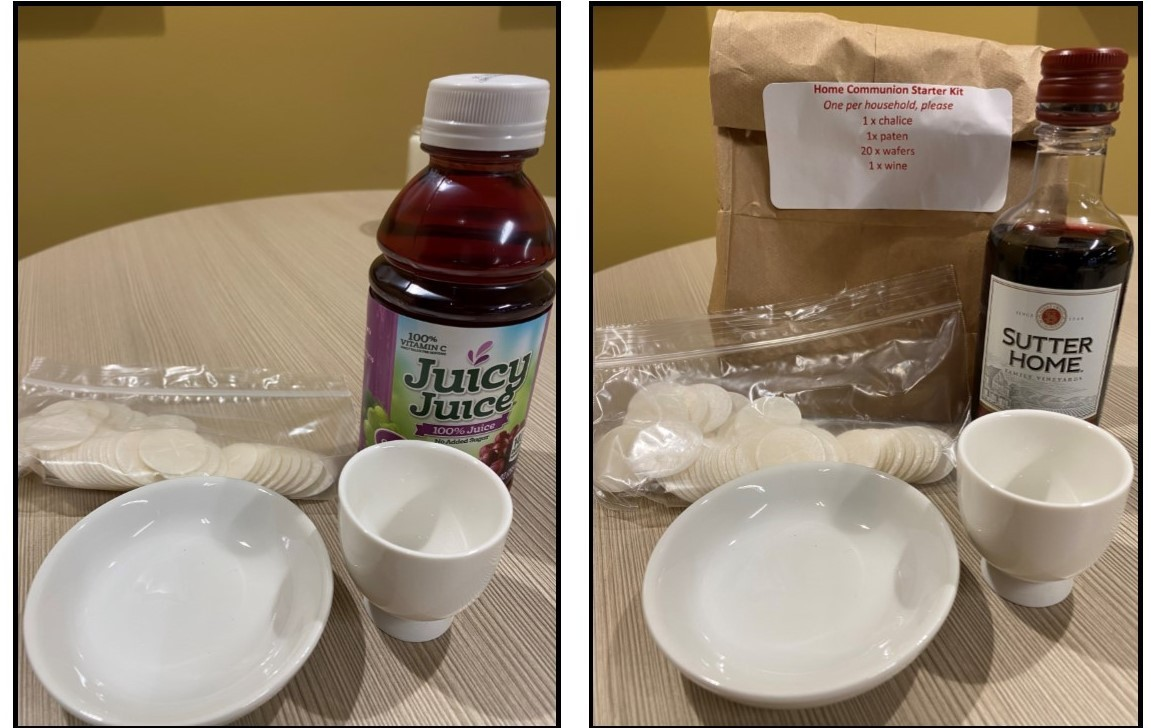Home Communion Kits
If you need to replenish your communion supplies, please stop by the church during office hours, Monday - Friday 9:00 am - 1:00 pm. If you need to come by after these hours, please call the church office at 815-398-3557. Watch this short video from Pastor Chad or read more here.

 table div table+table+table+table+table+table+table+table+table+table+table+table+table+table+table+table+table+table+table+table+table+table+table+table+table+table+table+table+table+table+table+table+table+table div table{width:100%;padding:0}table div table+table+table+table+table+table+table+table+table+table+table+table+table+table+table+table+table+table+table+table+table+table+table+table+table+table+table+table+table+table+table+table+table+table div table img{width:96.23%;padding:0;float:none}table div table+table+table+table+table+table+table+table+table+table+table+table+table+table+table+table+table+table+table+table+table+table+table+table+table+table+table+table+table+table+table+table+table+table div table td{width:100%;padding:0 1.88% 18px}/* styles */St. Mark 60
St. Mark 60 event for May will be making and delivering 60 baskets. When we moved to Rockford, the kids in our neighborhood made and delivered May baskets. What a sweet tradition. Have you ever made or received a May basket? Would you be available to help “deliver” May baskets? We would have them ready for you on Friday, April 30th. They could be delivered Friday or Saturday. Please sign-up and say you’ll help! melinda@stmarklc.com or call the church office.

 table div table+table+table+table+table+table+table+table+table+table+table+table+table+table+table+table+table+table+table+table+table+table+table+table+table+table+table+table+table+table+table+table+table+table+table+table div table{width:100%;padding:0}table div table+table+table+table+table+table+table+table+table+table+table+table+table+table+table+table+table+table+table+table+table+table+table+table+table+table+table+table+table+table+table+table+table+table+table+table div table img{width:96.23%;padding:0;float:none}table div table+table+table+table+table+table+table+table+table+table+table+table+table+table+table+table+table+table+table+table+table+table+table+table+table+table+table+table+table+table+table+table+table+table+table+table div table td{width:100%;padding:0 1.88% 18px}/* styles */St. Mark Mobile App!
Download our free mobile app on the app store or google play. If you are having trouble downloading or have any questions, please call Jill Davenport at 815-218-3403 or email jill@stmarklc.com, she will help you through the process. This is different than our church directory app. We encourage you to download the app. It's about getting involved and staying connected.

Click on this link for information on how to download the app!

 table div table+table+table+table+table+table+table+table+table+table+table+table+table+table+table+table+table+table+table+table+table+table+table+table+table+table+table+table+table+table+table+table+table+table+table+table+table+table div table{width:100%;padding:0}table div table+table+table+table+table+table+table+table+table+table+table+table+table+table+table+table+table+table+table+table+table+table+table+table+table+table+table+table+table+table+table+table+table+table+table+table+table+table div table img{width:96.23%;padding:0;float:none}table div table+table+table+table+table+table+table+table+table+table+table+table+table+table+table+table+table+table+table+table+table+table+table+table+table+table+table+table+table+table+table+table+table+table+table+table+table+table div table td{width:100%;padding:0 1.88% 18px}/* styles */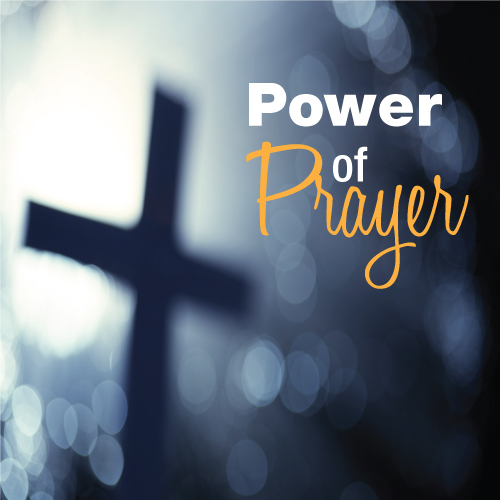St. Mark Prayer Chain
Would you like to be a part of the St. Mark prayer chain? Prayer requests come into the church office weekly. As we receive prayer requests we would send out an e-mail and ask that you pray daily for the persons mentioned in the requests. All requests are to remain confidential. Please e-mail melinda@stmarklc.com if you would like to participate in the prayer chain. “Be anxious for nothing, but in everything by prayer and supplication, with thanksgiving, let your requests be made known to God.” Colossians 4:2.

 table div table+table+table+table+table+table+table+table+table+table+table+table+table+table+table+table+table+table+table+table+table+table+table+table+table+table+table+table+table+table+table+table+table+table+table+table+table+table+table+table div table{width:100%;padding:0}table div table+table+table+table+table+table+table+table+table+table+table+table+table+table+table+table+table+table+table+table+table+table+table+table+table+table+table+table+table+table+table+table+table+table+table+table+table+table+table+table div table img{width:96.23%;padding:0;float:none}table div table+table+table+table+table+table+table+table+table+table+table+table+table+table+table+table+table+table+table+table+table+table+table+table+table+table+table+table+table+table+table+table+table+table+table+table+table+table+table+table div table td{width:100%;padding:0 1.88% 18px}/* styles */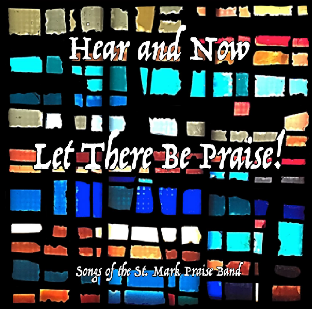St. Mark Praise Band Album Now Available

Good news! The St. Mark praise band, Hear and Now, has just released their first Album! It is a collection of original pieces and a few classic old favorites and is available as a compact disc (CD) or digitally thru Band Camp.

The CD’s will be available for pick up at the church in the hallway. A \$10 donation is asked to help offset the cost of producing the Album.

Digital copies are available at the following link. A suggested price of \$9 hearandnow1.bandcamp.com

 table div table+table+table+table+table+table+table+table+table+table+table+table+table+table+table+table+table+table+table+table+table+table+table+table+table+table+table+table+table+table+table+table+table+table+table+table+table+table+table+table+table+table div table{width:100%;padding:0}table div table+table+table+table+table+table+table+table+table+table+table+table+table+table+table+table+table+table+table+table+table+table+table+table+table+table+table+table+table+table+table+table+table+table+table+table+table+table+table+table+table+table div table img{width:96.23%;padding:0;float:none}table div table+table+table+table+table+table+table+table+table+table+table+table+table+table+table+table+table+table+table+table+table+table+table+table+table+table+table+table+table+table+table+table+table+table+table+table+table+table+table+table+table+table div table td{width:100%;padding:0 1.88% 18px}/* styles */For the safety of all our members, and in keeping with our calling as Christians to love our neighbors, groups that meet on-site must follow these guidelines. Groups that meet off-site strongly encouraged to follow these to the best of their ability. The health and welfare of our members and visitors is our number one priority in resuming all activities at St. Mark Lutheran Church. When 100% of attendees are fully vaccinated against COVID-19, certain precautions are not required. Small group leaders are responsible for determining if their group is fully vaccinated and able to forego these guidelines. See the link below for the full guidelines, the church council and pastors have put in place. Thank you.
Click here Guidelines for Small Groups at St. Mark

 table div table+table+table+table+table+table+table+table+table+table+table+table+table+table+table+table+table+table+table+table+table+table+table+table+table+table+table+table+table+table+table+table+table+table+table+table+table+table+table+table+table+table+table+table div table{width:100%;padding:0}table div table+table+table+table+table+table+table+table+table+table+table+table+table+table+table+table+table+table+table+table+table+table+table+table+table+table+table+table+table+table+table+table+table+table+table+table+table+table+table+table+table+table+table+table div table img{width:96.23%;padding:0;float:none}table div table+table+table+table+table+table+table+table+table+table+table+table+table+table+table+table+table+table+table+table+table+table+table+table+table+table+table+table+table+table+table+table+table+table+table+table+table+table+table+table+table+table+table+table div table td{width:100%;padding:0 1.88% 18px}/* styles */## Our St. Mark members:

Jan Stringer, Adleigh Siemans, Lorine Kortus, Russ Oellig, Linda Crooks, Cathy Trost, Jan Cotter, Roger Lind, Diane Swanson, Billie Reinhold, Sherri Lindquist, Bill Jennings, Tim Panjkovich, Bette Patterson, David Young, Keith Haggestad.

## Family and friends of St. Mark members:

Conrad Semmelroth, David & Alice Borden, Nici King, Vicki Kalnins, Kris & Jack O'Brien, Kate Cuccaro, Debbie Adams, Susan Vincent, Wanda Adamson, Milane Bagel, Janet Webster, Rae Ann McIntire, Linda Johnson, Eva Love Sherbondy, Norma Comstock, Carolyn King, Ashton Henley.

## Those serving in the military and their families:

Hunter Haggestad, Tristan Davenport, Scot Hornick, Bailey Panjkovich, Xander Gehrke, Jim Tammen, Brandon Ballenger, Taylor Eversole, Dean Barron.

Strengthen and encourage those in public health services and in the medical profession: care-givers, nurses, attendants, doctors, all who commit themselves to care for the sick and their families.

 table div table+table+table+table+table+table+table+table+table+table+table+table+table+table+table+table+table+table+table+table+table+table+table+table+table+table+table+table+table+table+table+table+table+table+table+table+table+table+table+table+table+table+table+table+table+table+table div table{width:100%;padding:0}table div table+table+table+table+table+table+table+table+table+table+table+table+table+table+table+table+table+table+table+table+table+table+table+table+table+table+table+table+table+table+table+table+table+table+table+table+table+table+table+table+table+table+table+table+table+table+table div table img{width:96.23%;padding:0;float:none}table div table+table+table+table+table+table+table+table+table+table+table+table+table+table+table+table+table+table+table+table+table+table+table+table+table+table+table+table+table+table+table+table+table+table+table+table+table+table+table+table+table+table+table+table+table+table+table div table td{width:100%;padding:0 1.88% 18px}/* styles *//* styles */ Office Hours Monday through Friday 9:00 A.M. - 4:00 P.M. Telephone: 815-398-3557 Publications St. Mark Memos, the weekly e-newsletter, is distributed each Thursday. The deadline for submission is Monday by 3:00 P.M. Please click to send submissions. Sermons Online Miss a Sunday at St. Mark? Please visit our website and watch the sermons online. Please click to watch.